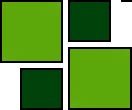ISSN 1842-4562 Member of DOAJ

# Analytical and Numerical Studies of Perturbed Renewal Equations with Multivariate Non-Polynomial Perturbations

## Abstract

The object of study is a model of nonlinearly perturbed continuous-time renewal equation with multivariate non-polynomial perturbations. The characteristics of the distribution generating the renewal equation are assumed to have expansions in a perturbation parameter with respect to a non-polynomial asymptotic. Exponential asymptotics for such a model as well as their applications are given. Numerical studies are performed to gain insights into the asymptotical results.

(top)